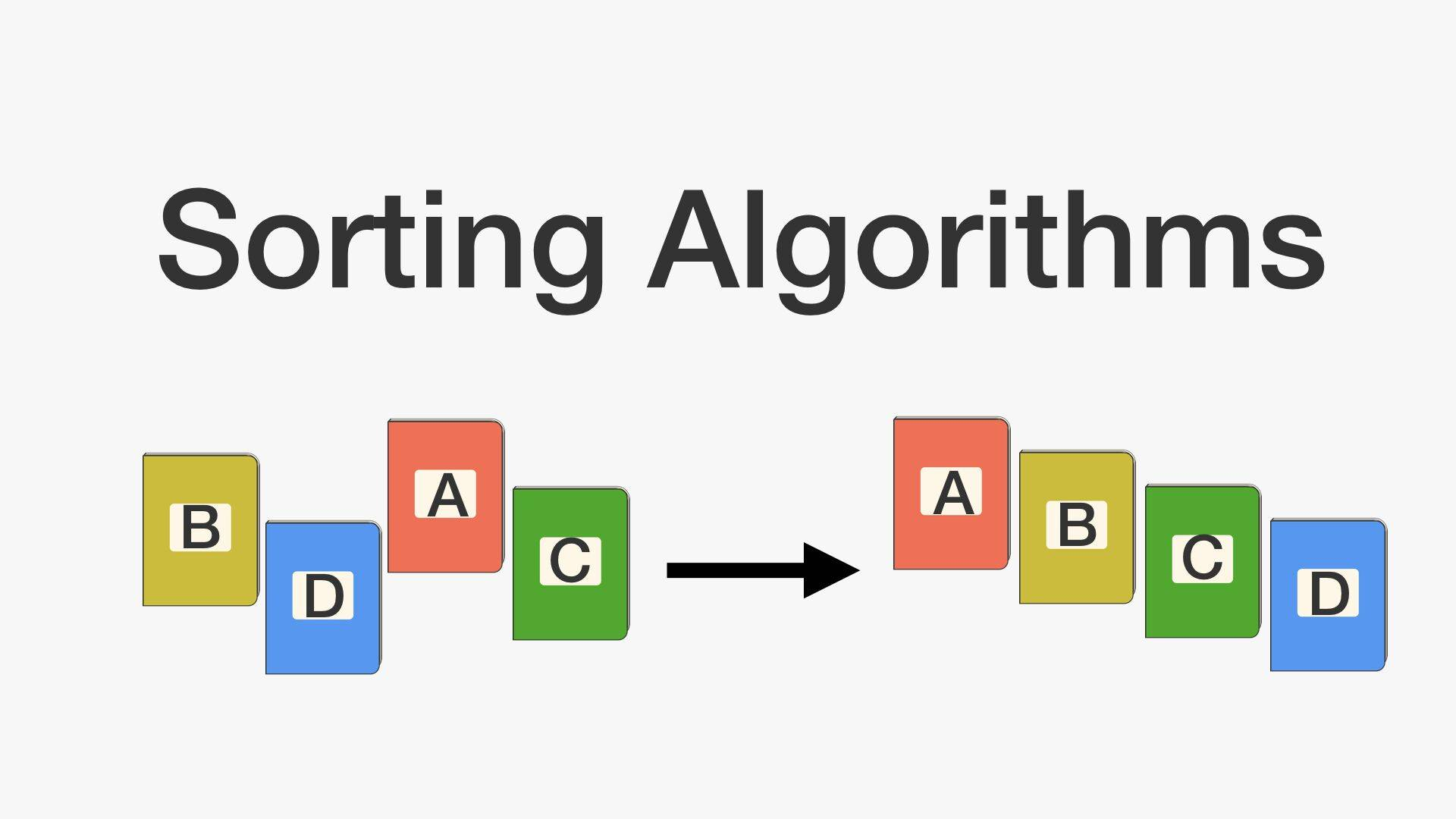# 基礎電腦科學：排序（sorting）演算法入門上# 選擇排序法

1. 給定一個數字組合和初始最小值位值（一開始 index 為 0）
2. 經過第一輪每個數字和最小值比較，將取出的最小值和第一個數字位置對調（選擇最小的值）
3. 接著除了第一個已排序好的數字外，其餘數字持續最小值比較（index 為 1），若有更小值則和 index 為 1（第二個）值互換
4. 持續進行比較到能比較的值只剩下一個，則由小到大的排序完成

1. 第一次排序後：2 [4, 11, 9, 7]：2 比 9 小，所以互換位置

2. 第二次排序後：2, 4, [11, 9, 7]，4 為初始最小值，沒有可以互換的值

3. 第三次排序後：2, 4, [11, 9, 7]，11 為初始最小值，7 是右邊比 11 小的最小值，兩者互換

4. 第四次排序後：2, 4, 7, [9, 11]，9 為初始最小值，沒有可以互換的值

5. 第五次排序後：2, 4, 7, 9, ，11 為初始最小值，沒有可以互換的值

6. 最後排序結果：2, 4, 7, 9, 11

def selection_sort(num_list):
"""
:param num_list: a list want to sort
"""
num_list_length = len(num_list)
for i in range(0, num_list_length):
min_num_index = i
for j in range(i + 1, num_list_length):
if num_list[min_num_index] > num_list[j]:
min_num_index = j
num_list[min_num_index], num_list[i] = num_list[i], num_list[min_num_index]

return num_list

if __name__ == '__main__':
num_list = [9, 4, 11, 2, 7]
print('選擇排序前：{}'.format(num_list))
print('選擇排序後：{}'.format(selection_sort(num_list)))


# 插入排序法

1. 給定一個數字組合並假設第一個值成為一已排序數列
2. 經過第一輪每個數字和已排序數列比較，將值插入已排序數列對應位置
3. 直到最後一個數字也已插入已排序列中則排序結束

1. 第一步插入 9 後：, 4, 11, 2, 7

2. 第二步插入 4 後：[4, 9], 11, 2, 7

3. 第三步插入 11 後：[4, 9, 11], 2, 7

4. 第四步插入 9 後：[2, 4, 9, 11], 7

5. 最後排序結果：[2, 4, 7, 9, 11]

def insert_sort(num_list):
"""
:param num_list: a list want to sort
"""
num_list_length = len(num_list)
for i in range(1, num_list_length):
insert_value = num_list[i]
j = i - 1
while j >= 0:
if num_list[j] > insert_value:
num_list[j + 1] = num_list[j]
num_list[j] = insert_value
j -= 1

return num_list

if __name__ == '__main__':
num_list = [9, 4, 11, 2, 7]
print('插入排序前：{}'.format(num_list))
print('插入排序後：{}'.format(insert_sort(num_list)))


# 氣泡排序法

1. 第一輪交換後：4, 9, 2, 7, 11

2. 第二輪交換後：4, 2, 7, 9, 11

3. 第三輪交換後：2, 4, 7, 9, 11

4. 最後排序結果：2, 4, 7, 9, 11

def bubble_sort(num_list):
"""
:param num_list: a list want to sort
"""
list_length = len(num_list)
for i in range(0, list_length):
for j in range(i + 1, list_length):
if num_list[i] > num_list[j]:
num_list[i], num_list[j] = num_list[j], num_list[i]
return num_list

if __name__ == '__main__':
num_list = [9, 4, 11, 2, 7]
print('氣泡排序前：{}'.format(num_list))
print('氣泡排序後：{}'.format(bubble_sort(num_list)))


# 參考文件

（image via pandorafmsbrilliant

@kdchang 文藝型開發者，夢想是做出人們想用的產品和辦一所心目中理想的學校。A Starter & Maker. JavaScript, Python & Arduino/Android lover.:)